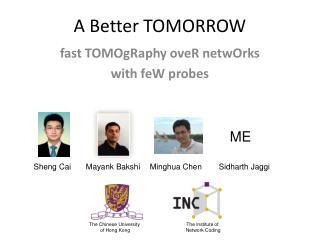DownloadDownload PresentationA Better TOMORROW

# A Better TOMORROW

Télécharger la présentation## A Better TOMORROW

- - - - - - - - - - - - - - - - - - - - - - - - - - - E N D - - - - - - - - - - - - - - - - - - - - - - - - - - -
##### Presentation Transcript

1. A Better TOMORROW ME fast TOMOgRaphy oveR netwOrks with feW probes Sheng Cai Mayank Bakshi Minghua Chen Sidharth Jaggi The Chinese University of Hong Kong The Institute of Network Coding

2. FRANTIC ME Fast Reference-based Algorithm for Network Tomography vIa Compressive Sensing Sheng Cai Mayank Bakshi Minghua Chen Sidharth Jaggi The Chinese University of Hong Kong The Institute of Network Coding

3. Computerized Axial Tomography (CAT scan)

4. Tomography y = Tx Estimate x given y and T

5. Network Tomography • Transform T: • Network connectivity matrix (known a priori) • Measurements y: • End-to-end packet delays • Infer x: • Link congestion Hopefully “k-sparse” Compressive sensing? • Challenge: • Matrix T “fixed” • Idea: • “Mimic” random matrix

6. 1. Better CS [BJCC12] “SHO-FA”

7. 1. Better CS [BJCC12] “SHO-FA” O(k) measurements, O(k) time

8. SHO(rt)-FA(st)O(k) meas., O(k) steps

9. 1. Better CS [BJCC12] “SHO-FA” Need “sparse & random” matrix T

10. SHO-FA A d=3 ck n

11. 2. Better mimicking of desired T

12. Node delay estimation

13. Node delay estimation

14. Node delay estimation

15. Edge delay estimation

16. Idea 2: “Loopy” measurements , • Fewer measurements • Arbitrary packet injection/ • reception • Not just 0/1 matrices (SHO-FA)

17. SHO-FA + Cancellations + Loopy measurements • n = |V| or |E| • M = “loopiness” • k = sparsity • Measurements: O(k log(n)/log(M)) • Decoding time: O(k log(n)/log(M)) • General graphs, node/edge delay estimation • Path delay: O(DnM/k) • Path delay: O(D’M/k) (Steiner trees) • Path delay: O(D’’M/k) (“Average” Steiner trees) • Path delay: ??? (Graph decompositions)

18. 1. Graph-Matrix

19. 2. (Most) x-expansion ≥2|S| |S|

20. Decoding – Leaf Check(1-Passed)

21. m ? n m<n

22. Compressive sensing ? m ? n k k ≤ m<n

23. Robust compressive sensing ? e z y=A(x+z)+e Approximate sparsity Measurement noise

24. Apps: 1. Compression W(x+z) x+z BW(x+z) = A(x+z) M.A. Davenport, M.F. Duarte, Y.C. Eldar, and G. Kutyniok, "Introduction to Compressed Sensing,"in Compressed Sensing: Theory and Applications, Cambridge University Press, 2012.

25. Apps: 2. Network tomography Weiyu Xu; Mallada, E.; Ao Tang; , "Compressive sensing over graphs," INFOCOM, 2011 M. Cheraghchi, A. Karbasi, S. Mohajer, V.Saligrama: Graph-Constrained Group Testing. IEEE Transactions on Information Theory 58(1): 248-262 (2012)

26. Apps: 3. Fast(er) Fourier Transform H. Hassanieh, P. Indyk, D. Katabi, and E. Price. Nearly optimal sparse fourier transform. In Proceedings of the 44th symposium on Theory of Computing (STOC '12). ACM, New York, NY, USA, 563-578.

27. Apps: 4. One-pixel camera http://dsp.rice.edu/sites/dsp.rice.edu/files/cs/cscam.gif

28. y=A(x+z)+e

29. y=A(x+z)+e

30. y=A(x+z)+e

31. y=A(x+z)+e

32. y=A(x+z)+e (Information-theoretically) order-optimal

33. (Information-theoretically) order-optimal • Support Recovery

34. SHO(rt)-FA(st)O(k) meas., O(k) steps

35. SHO(rt)-FA(st)O(k) meas., O(k) steps

36. SHO(rt)-FA(st)O(k) meas., O(k) steps

37. 1. Graph-Matrix A d=3 ck n

38. 1. Graph-Matrix A d=3 ck n

39. 1. Graph-Matrix

40. 2. (Most) x-expansion ≥2|S| |S|

41. 3. “Many” leafs L+L’≥2|S| ≥2|S| |S| 3|S|≥L+2L’ L≥|S| L+L’≤3|S| L/(L+L’) ≥1/2 L/(L+L’) ≥1/3

42. 4. Matrix

43. Encoding – Recap. 0 1 0 1 0

44. Decoding – Initialization